# Find the corresponding graphs for the distance-time graphs

• chwala
Gold Member
Homework Statement
match the distance- time graphs to their corresponding velocity - time graphs
Relevant Equations
kinematics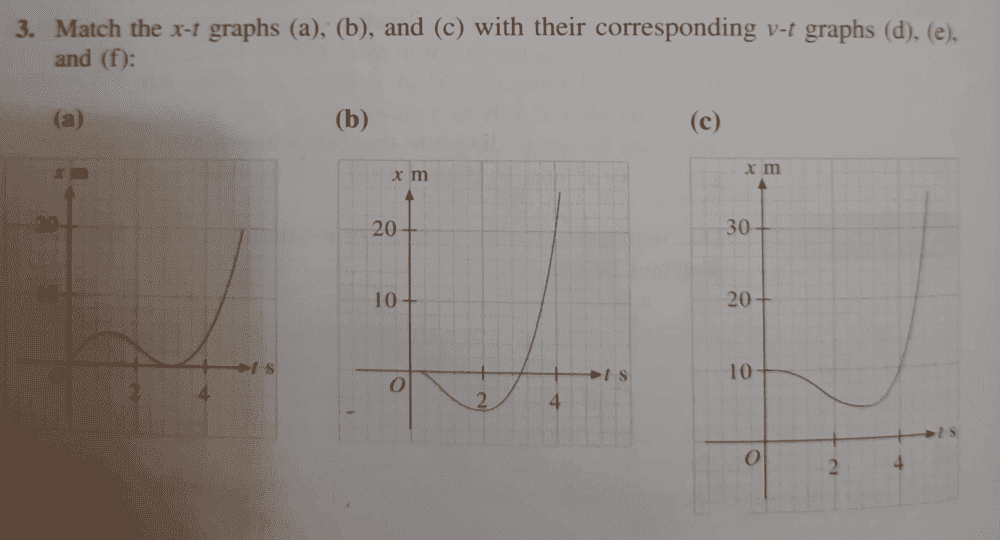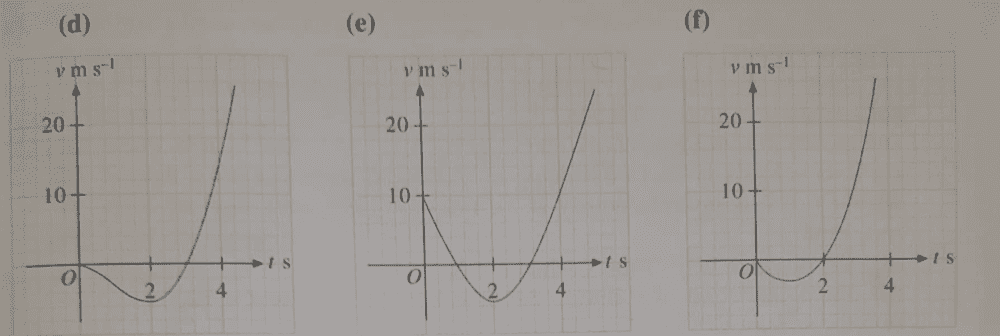now there is something i am not getting here, consider for instance graph ##a##,
analyzing this graph i can say as follows;
between time ##0≤ t<1, ## the velocity is increasing...
at time ##t=1##, also reffered to as being intantaneously at rest, at this point we have acceleration ##a=0##,
between time##1< t<3 ##, the velocity is decreasing...
at the point ##t=3##, we have ##a=0##,
between time ##3< t<5 ##,velocity is increasing...

is my analysis correct?

lets start with the graph a. Now if my analysis is correct, which corresponding graph will suffice?
i would expect that if velocity is increasing between time ##0≤ t<1,## then acceleration should equally be increasing, and i cannot see which graph reflects that...

Homework Helper
consider for instance graph ##a##,
analyzing this graph i can say as follows;
between time ##0≤ t<1, ## the velocity is increasing... ##\qquad## (1)
at time ##t=1##, also reffered to as being intantaneously at rest, ##\qquad## (2)
at this point we have acceleration ##a=0##,
between time##1< t<3 ##, the velocity is decreasing...
at the point ##t=3##, we have ##a=0##,
between time ##3< t<5 ##,velocity is increasing...

is my analysis correct?
I propose you reconsider:
(1) the position ##x## is increasing, but it is going at a lower and lower rate. What does that say about the velocity, which is the rate of change of the position ?

(2) "at rest" says what about the velocity ?

##\ ##

Gold Member
to answer part ##(2)##, at rest, literally means that the velocity at that point ##=0##, i am still trying to figure out part##(1)##. I still do not understand what you mean by lower and lower rate. The rate that you are 'reffering' to can simply be called velocity. The velocity i.e the gradient value is positive at that particular interval that i mentioned, hence use of my term increasing...

Gold Member
ok, i can see that from
 x= 3 4.1 4.2 4 3 t=0.4 0.8 1.2 1.6 2

ok Bvu sir, i can see that the rate of change i.e velocity is reducing i.e ##[7.5, 5.125, 3.5, 2.5, 1.5]## m/s...

Last edited:
Gold Member
ok, i can see that from
 x= 3 4.1 4.2 4 3 t=0.4 0.8 1.2 1.6 2

the rate of change i.e velocity is reducing i.e ##[7.5, 5.125, 3.5, 2.5, 1.5]## m/s...

approah 2
consider the velocity time graph ##e##, at time ##t=0## and ##t=1## the graph at this points are represented by a straight line, therefore the distance traveled betwen these two points, ##x= 0.5 ×1×10= 5m## which corresponds only with distance- time graph ##(a)## having co ordinates ##(x,t)=(1,5)##.
am i right? therefore for this case, ##a→e##

Note textbook answer are ##[a→e, b→f, c→d]##

Last edited:
Homework Helper
Dear Chwala,

This is an exercise in differentiation and integration, both very important for physics.

Differentiation

You know that the rate of change in position is the velocity: ##v = \displaystyle {\Delta x\over \Delta t} ##

When things change continuously, this becomes a differential (instantaneous velocity):$$v(t) ={dx(t)\over dt}$$
This brings you from graphs of ##\ x(t)\ ## to graphs of ##\ v(t)\ ## because ##\ \displaystyle {dx(t)\over dt} ## is the slope of the ##x(t)## graph at time ##t##. You take a transparent ruler and look for tangents.
For example in figure a) the interesting ones are: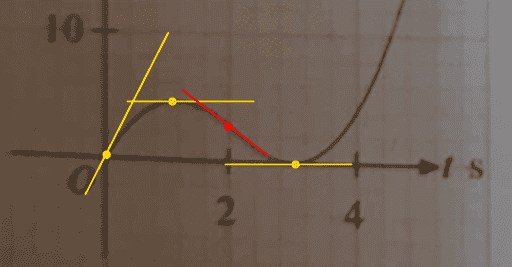(sorry for the quality)
• at ##t=0## the yellow line with a slope of 10 m/s.
• at ##t=1## and ##t=3## the orange lines with slope 0 ##\Rightarrow\ v(t)=0## m/s.
• and, finally and very interesting: at ##t=2## you have an inflexion point wth slope -1 m/s.
Inflection points are very helpful: at such a point the curve changes from convex to concave (or vice versa), so the slope is at a minimum (or maximum).​
With these four characterizing points it is very easy to pick the right ##\ v(t)\ ## graph !

Integration

From $$v(t) ={dx(t)\over dt}$$ we go to (mathematicians won't like this, but we doit without hesitation) $$v \Delta t =\Delta x\quad\Rightarrow\quad dx(t) = v(t) dt \quad\Rightarrow\quad x-x_0 = \int dx(t) =\int v(t) dt$$And this brings you from graphs of ##v(t)## to graphs of ##x(t)##.

The dotted items for integration in reverse: [edit:sorry, mistake in first edition]

The dotted items used for differentiation we reverse for integration :
• the y-axis crosssings give you extrema: ##v(t) = ## zero
• Extrema give you inflexion points
What you don't get is ##x_0## : the constant of integration can not be found from the integrand ##v(t)##.​
So in that respect integration deserves a bit more attention than differentiation. You may need to draw a line with a given slope but unknown intercept at some point(s). The most logical candidate is at ##t=0##.

As an example I could do this for figure e) but by now we know where that leads us, don't we ?
Instead, why don't you try figure d) in this manner to see if I have actually been helpful in this.

(It is a great pity you already have the answers, it distorts unbiased thinking).

##\ ##

Last edited:
•chwala
Gold Member
Dear Chwala,

This is an exercise in differentiation and integration, both very important for physics.

Differentiation

You know that the rate of change in position is the velocity: ##v = \displaystyle {\Delta x\over \Delta t} ##

When things change continuously, this becomes a differential (instantaneous velocity):$$v(t) ={dx(t)\over dt}$$
This brings you from graphs of ##\ x(t)\ ## to graphs of ##\ v(t)\ ## because ##\ \displaystyle {dx(t)\over dt} ## is the slope of the ##x(t)## graph at time ##t##. You take a transparent ruler and look for tangents.
For example in figure a) the interesting ones are:

(sorry for the quality)
• at ##t=0## the yellow line with a slope of 10 m/s.
• at ##t=1## and ##t=3## the orange lines with slope 0 ##\Rightarrow\ v(t)=0## m/s.
• and, finally and very interesting: at ##t=2## you have an inflexion point wth slope -1 m/s.
Inflection points are very helpful: at such a point the curve changes from convex to concave (or vice versa), so the slope is at a minimum (or maximum).​
With these four characterizing points it is very easy to pick the right ##\ v(t)\ ## graph !

Integration

From $$v(t) ={dx(t)\over dt}$$ we go to (mathematicians won't like this, but we doit without hesitation) $$v \Delta t =\Delta x\quad\Rightarrow\quad dx(t) = v(t) dt \quad\Rightarrow\quad x-x_0 = \int dx(t) =\int v(t) dt$$And this brings you from graphs of ##v(t)## to graphs of ##x(t)##. The dotted items for integration in reverse:
• The y-axis crosssings give you extrema: ##v(t) = ## zero
• Extrema give you inflexion points
What you don't get is ##x_0## : the constant of integration can not be found from the integrand ##v(t)##.​
So in that respect integration deserves a bit more attention than differentiation. You may need to draw a line with a given slope but unknown intercept at some point(s). The most logical candidate is at ##t=0##.

As an example I could do this for figure e) but by now we know where that leads us, don't we ?
Instead, why don't you try figure d) in this manner to see if I have actually been helpful in this.

(It is a great pity you already have the answers, it distorts unbiased thinking).

##\ ##
Thanks for your time on this,...looks like it requires some indepth thinking...I guess differentiation would be easier than integration going by what I see from your approach nevertheless I will take your advise, form the tangents and see whether I could get the ##x## function ...

Homework Helper
 I forgot one that doesn't appear in this exercise. So, for completeness:
• In differentiation a straight section means a constant derivative
• When integrating vice versa
(One can partially link this to the inflexion point where the derivative is "instantaneously constant")

•chwala
Gold Member
so if we consider graph 3.e, by considering a straight line ...passing through the points ##(0,0)## and ##(0.8,2)## , we shall have,
##x=∫2.5t dt= 1.25t^2+k##

Last edited:
Gold Member
 I forgot one that doesn't appear in this exercise. So, for completeness:
• In differentiation a straight section means a constant derivative
• When integrating vice versa
(One can partially link this to the inflexion point where the derivative is "instantaneously constant")

i want to understand the graph 3.e ...ok i want to get this right, so in integration i should be using the constant derivative to get the straight section(distance function) right? by considering the points ##(0,0)## and ##(0.8,2)## i am getting a gradient of ##2.5##, is this correct...therefore is this the value that i am integrating?

Homework Helper
so if we consider graph 3.(e), by considering a straight line ...passing through the points ##(0.0)## and ##(0.8,2)## , we shall have,
##x=∫2.5t dt= 1.25t^2+k##
Remember that you want to integrate the graph of ##v(t)##.

• the y-axis crosssings give you extrema: ##v(t) = ## zero
• Extrema give you inflexion points
What you don't get is ##x_0## : the constant of integration can not be found from the integrand ##v(t)##.

I do not see the point ##(0,0)## lying on the graph ! Nor the point ##(0.8, 2)## !Gold Member
ok btwn ##t=0## and ##t=1##, we shall have,
##v=∫-10 dt= -10t +k## applying the conditions, ##(0,10)##,
we have ##v=-10t +10##
therefore, ##x=∫vdt=\int_0^1[-10t+10]\,dt=5m##

Last edited:
Homework Helper
I tried to make clear to you that you can not obtain ##x_0## from a ##v(t)## plot, because constants disappear when differentiating. So the best you can get is ##\Delta x##.

You now treat the first second of (e) as a straight line, which is ok, but clearly an approximation.[edit sorry: ##x = 0## does not mean crosssing the y-axis --- Why not start with finding the y-axis crossings]

Why not start with finding the time-axis crossings of the ##v(t)## plot -- they provide the moments when the plot of ##x(t)## is horizontal. Namely at ##t-1## s and ##t= 3## s (orange points in the picture below).

At ##t=1## s ##v ## changes from positive (forward) to negative (backward), so that is a maximum in the ##x(t)## plot.
At ##t=3## s (a little more actually), ##v ## changes from negative (backward) to positive (forward), which means that's a minimum in the ##x(t)## plot.

Then the next easy one is the minimum in ##v(t)## at ##t = 2## s (red) : an inflexion point in the ##x(t)## plot.

If these three are not enough to identify the corresponding ##x(t)## plot, you can still make a decisive distinction with the ##v(0) = 10## (left yellow point)which is the slope of the ##x(t)## plot at ##t=0##, thereby discarding possibilities (b) and (c)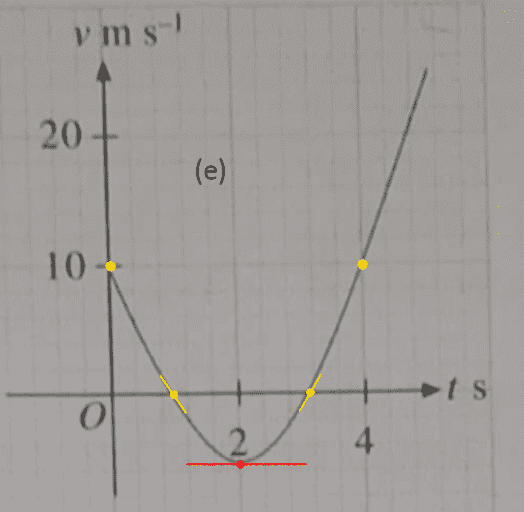Any questions?
If not, can you analyse picture (f) ?

Last edited:
•chwala
Gold Member
thanks bvu, phew! sweating here, so basically you are implying that we can look at the behaviour of the gradients on the v-t graph to realize the corresponding maxima and minima points on the distance -time graph and generally come to some deduction...i think i am a bit confused here because the approach that i had was by considering the rate of change on the v-t graph being accelaration function...

Gold Member
I tried to make clear to you that you can not obtain ##x_0## from a ##v(t)## plot, because constants disappear when differentiating. So the best you can get is ##\Delta x##.

You now treat the first second of (e) as a straight line, which is ok, but clearly an approximation.

Why not start with finding the y-axis crossings -- they provide the moments when the plot of ##x(t)## is horizontal. Namely at ##t-1## s and ##t= 3## s (orange points in the picture below).

At ##t=1## s ##v ## changes from positive (forward) to negative (backward), so that is a maximum in the ##x(t)## plot.
At ##t=3## s (a little more actually), ##v ## changes from negative (backward) to positive (forward), which means that's a minimum in the ##x(t)## plot.

Then the next easy one is the minimum in ##v(t)## at ##t = 2## s (red) : an inflexion point in the ##x(t)## plot.

If these three are not enough to identify the corresponding ##x(t)## plot, you can still make a decisive distinction with the ##v(0) = 10## (left yellow point)which is the slope of the ##x(t)## plot at ##t=0##, thereby discarding possibilities (b) and (c)

View attachment 278294

Any questions?
If not, can you analyse picture (f) ?

yep, let me analyse graph##f## with the y- crossings...i will check on this later in the day and post back...cheers

Homework Helper
so basically you are implying that we can look at the behaviour of the gradients on the v-t graph to realize the corresponding maxima and minima points on the distance -time graph
I should hope not ! I meant of course the axis crossings where ##v(t)=0##

-- I found it and corrected; sorry and thanks.

##\ ##

•chwala
Gold Member
Thanks Bvu, talk later in the day in regards to ##f##. Am running other errands...

Gold Member
I should hope not ! I meant of course the axis crossings where ##v(t)=0##

-- I found it and corrected; sorry and thanks.

##\ ##
ok, noted ...

Gold Member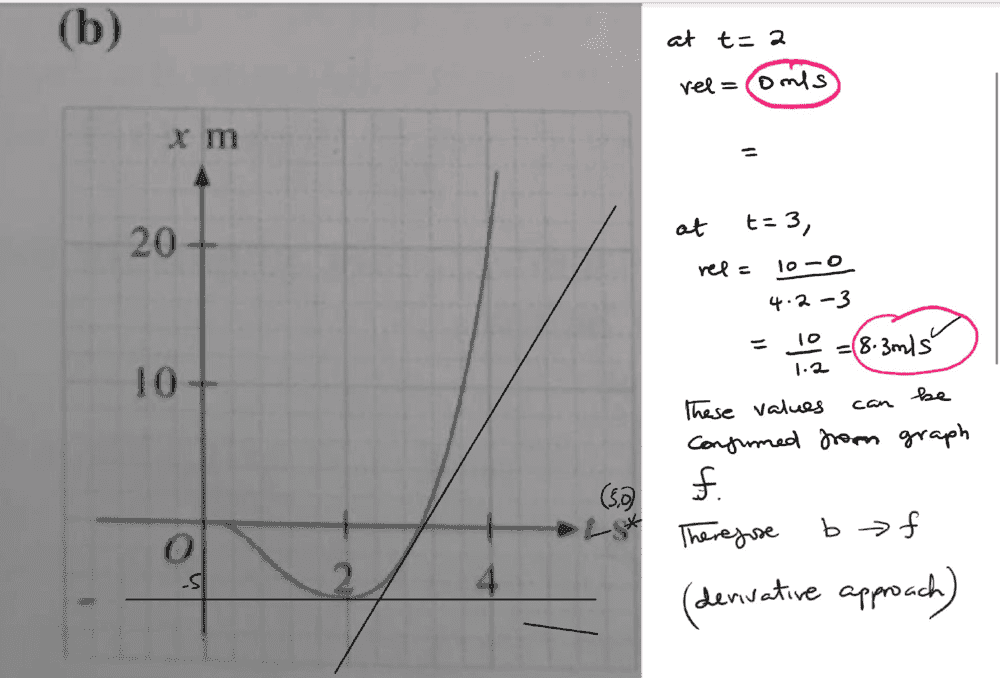i have used the derivative approach of drawing tangents...and clearly i can see that this graph ##b## will correspond to the ##v-t## graph## f##.
In this ##x-t## graph, kindly note that we are not able to draw tangents at value ##t=1##

Gold Member
View attachment 278324

i have used the derivative approach of drawing tangents...and clearly i can see that this graph ##b## will correspond to the ##v-t## graph## f##.
In this ##x-t## graph, kindly note that we are not able to draw tangents at value ##t=1##
if correct i will proceed to graph ##c## then use the reverse method ##v(t)=0## to match graphs ##e## and ##f## back to their corresponding ##x-t## graphs...

Homework Helper
Well done !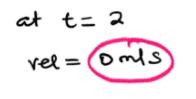I would draw the tangent at ##t=3## in (b) a little steeper: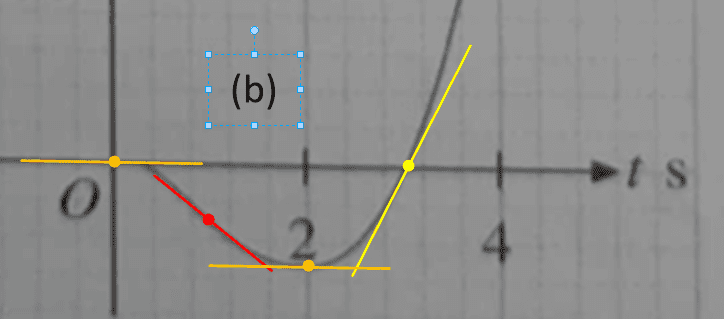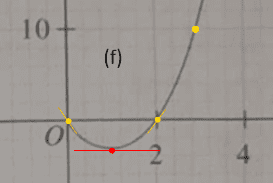In (f) you also have ##\ v=0\ ## at ##\ t=0\ ##, so the ##\ x(t)\ ## plot must take off horizontally. However, this does not help to distinguish between (b) and (c) , because both start the same way.

Furthermore: on (f) at ##t=1## you can conclude there must be an inflexion point in the ##x(t) \ ## graph. This does distinguish between (b) and (c) .

if correct i will proceed to graph c then use the reverse method ##\ v(t)=0\ ## to match graphs e and f back to their corresponding ##\ x−t \ ## graphs...
Excellent plan !

##\ ##

Gold Member
Well done !

View attachment 278325

I would draw the tangent at ##t=3## in (b) a little steeper:

In (f) you also have ##\ v=0\ ## at ##\ t=0\ ##, so the ##\ x(t)\ ## plot must take off horizontally. However, this does not help to distinguish between (b) and (c) , because both start the same way.

Furthermore: on (f) at ##t=1## you can conclude there must be an inflexion point in the ##x(t) \ ## graph. This does distinguish between (b) and (c) .

Excellent plan !

##\ ##

Thank you Bvu, i have learned a new concept here...

Homework Helper
So what's the story with (c) ?

•chwala
Gold Member
Let me look at this tomorrow...was a bit busy today...I will communicate then. Cheers

Gold Member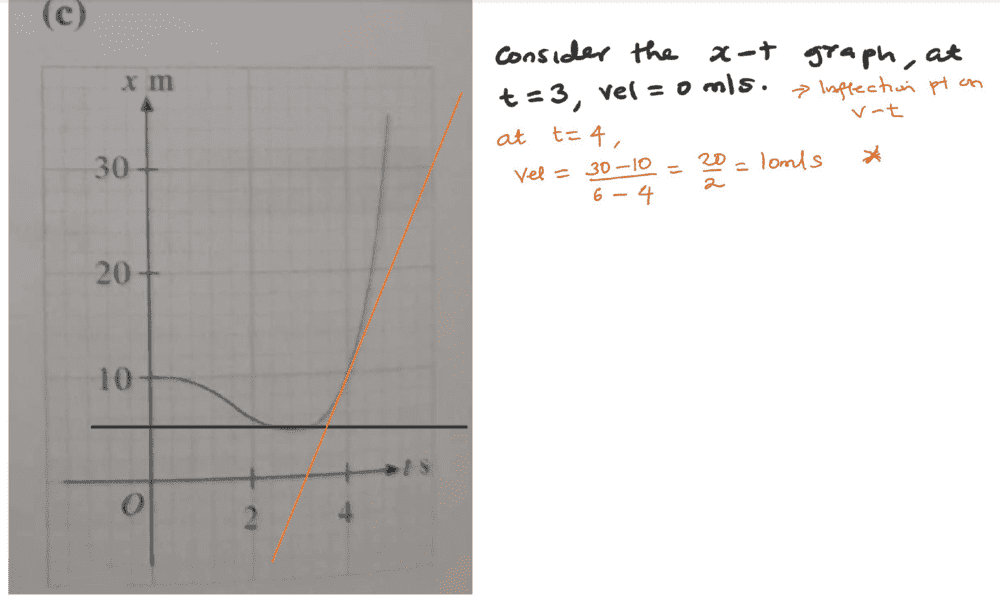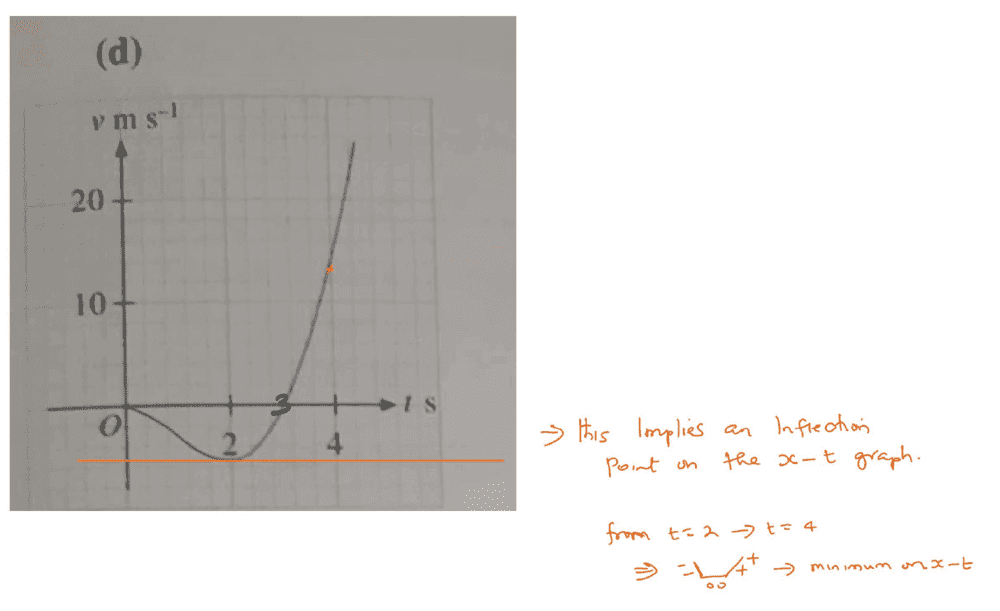this is what i came up with...
1. Considering the ##x−t## graph, at ##t=3,## velocity =##0m/s## agreeing with the ##v−t## graph.
2. I also tried drawing a tangent line on the ##x-t##graph at the point ##t=4##, ...there is a problem here, the values are not in sync...i am getting ##v(t)=10m/s## but correct value is ##v(t)=14m/s##
3. Analyzing the v−t graph, we have a stationary point at ##t=2## which implies an inflection on the ##x−t## graph
4. At the point ##t=2## to ##t=4## on the ##v-t## graph the graph changes from negative to positive, this implies a minimum point on the ##x−t## graph.

Homework Helper
Chwala said:
1. Considering the ##x−t## graph, at ##t=3,## velocity =##0## m/s, agreeing with the ##v−t## graph.
I agree. ##v=0## is therefore a point where ##v=0##, NOT an inflection point on ##v(t)##! Nor on ##x(t)##.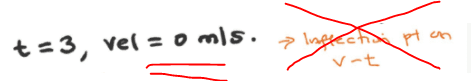(I see I misspelled inflection a few times -- so I learn from this thread too !)​

Chwala said:
2. I also tried drawing a tangent line on the ##x-t##graph at the point ##t=4##, ...there is a problem here, the values are not in sync...i am getting ##v(t)=10m/s## but correct value is ##v(t)=14m/s##
No reason for worry - the difference between ##\arctan 14## and ##\arctan 10## is only 2% .​

3. Analyzing the v−t graph, we have a stationary point at ##t=2## which implies an inflection on the ##x−t## graph
Excellent !

Chwala said:
4. At the point ##t=2## to ##t=4## on the ##v-t## graph the graph changes from negative to positive, this implies a minimum point on the ##x−t## graph.
To be precise: at ##t=3##. Very good too !

I think we have milked out the graphical stuff quite well and quite satisfactory by now. So I want to do something in another direction. Usually I tell posters to make a sketch if all I see is symbols and equations. This time it's all graphs and now I have a small challenge:

The graph (f) looks suspiciously like a plot of ##v(t) = 3.66 \,t \, (t-2) ##: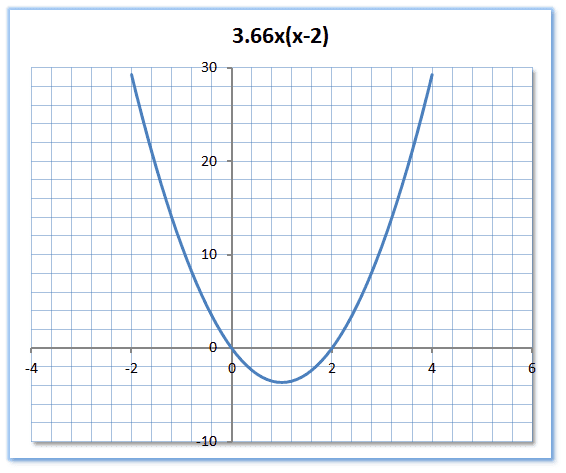So can you -- analytically -- tell me the equation for ##x(t) \ ## ?##\ ##

•chwala
Gold Member
I agree. ##v=0## is therefore a point where ##v=0##, NOT an inflection point on ##v(t)##! Nor on ##x(t)##.

(I see I misspelled inflection a few times -- so I learn from this thread too !)​

No reason for worry - the difference between ##\arctan 14## and ##\arctan 10## is only 2% .​

Excellent !

To be precise: at ##t=3##. Very good too !

I think we have milked out the graphical stuff quite well and quite satisfactory by now. So I want to do something in another direction. Usually I tell posters to make a sketch if all I see is symbols and equations. This time it's all graphs and now I have a small challenge:

The graph (f) looks suspiciously like a plot of ##v(t) = 3.66 \,t \, (t-2) ##:

So can you -- analytically -- tell me the equation for ##x(t) \ ## ?##\ ##
Ok I will work on this...communicate later..thanks bvu

Gold Member
I agree. ##v=0## is therefore a point where ##v=0##, NOT an inflection point on ##v(t)##! Nor on ##x(t)##.

(I see I misspelled inflection a few times -- so I learn from this thread too !)​

No reason for worry - the difference between ##\arctan 14## and ##\arctan 10## is only 2% .​

Excellent !

To be precise: at ##t=3##. Very good too !

I think we have milked out the graphical stuff quite well and quite satisfactory by now. So I want to do something in another direction. Usually I tell posters to make a sketch if all I see is symbols and equations. This time it's all graphs and now I have a small challenge:

The graph (f) looks suspiciously like a plot of ##v(t) = 3.66 \,t \, (t-2) ##:

So can you -- analytically -- tell me the equation for ##x(t) \ ## ?##\ ##
kindly clarify, i think you meant "find the equation for ##v(t)##"...as you had indicated earlier we cannot find ##x(t)##...as we do not have initial conditions...

Gold Member
let ##v=at^2-2at##
the turning point is at the point ##(1, -3)##,
therefore,
##-3=a-2a##
##→a=3##
therefore, ##v(t)=3t^2-6t##

Last edited:
Homework Helper
kindly clarify, i think you meant "find the equation for ##v(t)##"...as you had indicated earlier we cannot find ##x(t)##...as we do not have initial conditions...
No, I really meant

Find the equation for ##\ x(t)\ ##, given that the equation for ##\ v(t) \ ## is $$v(t) = 3.66 \,t \, (t-2) \ .$$As you correctly observe, you need one initial condition. Let that be ##\ x(0) = x_0\ ## with ##\ x_0 = 0 ## m.

Gold Member
then this should be easy,
##v(t)= 3t^2-6t##
##x(t)=∫vdt##
##x(t)=t^3-3t^2+k##
with condition, ##x(t=0.4)=0##
we have, ##x(t)=t^3-3t^2+0.416## as the required equation.

Homework Helper
then this should be easy,
Any particular reason you round off the 3.66 to 3 ?
And modify the condition ##\ x(0) = 0\ ## to ##\ x(0.4) = 0\ ## ?

Gold Member
Any particular reason you round off the 3.66 to 3 ?
And modify the condition ##\ x(0) = 0\ ## to ##\ x(0.4) = 0\ ## ?
I was working on equation ##f## and i considered its turning point to be points##(1,-3)## ... and on checking graph##b## i can see that when ##x=0, t=0.4##. If you want me to specifically use your ##v(t)## with turning points ##(1,-3.66)##then
we have ##v(t)= 3.66t^2-7.32t## integrating and applying initial condition, ##x(0)=0## yields,
##x(t)= 1.22t^3-3.66t^2## as the required equation.

Last edited:
Homework Helper
I was working on equation ##f## and i considered its turning point to be points##(1,-3)## ... and on checking graph##b## i can see that when ##x=0, t=0.4##.
If you want me to specifically use your ##v(t)## with turning points ##(1,-3.66)##then
The idea was that you would NOT look at any graphs, but simply do the integration. And indeed:
Chwala said:
we have ##v(t)= 3.66t^2-7.32t## integrating and applying initial condition, ##x(0)=0## yields,
##x(t)= 1.22t^3-3.66t^2## as the required equation.
Is perfect!
And, to top if off with a picture as prize: the graph looks exactly like figure (b)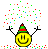: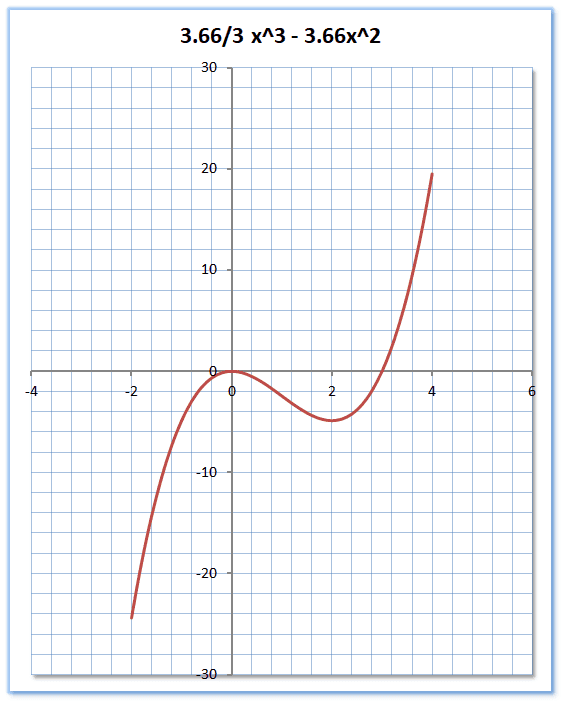Now there is really nothing more to be done with this exercise, so: on to the next !
##\ ##

•chwala
Gold Member
Thank you Bvu, i am assuming you are a Professor...you have great insight...thank you sir. I have learned on the behaviour of functions and their derivatives (considering the fact that the equation of the function has not been given) particularly on the aspect of moving from negative to positive for eg considering a function which implies the converse i.e positive-negative when it comes to its derived function. I also learned something on inflection points implying either a maximum or a minima on corresponding given functions.

Last edited: